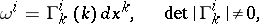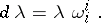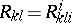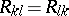# Equi-affine connection

(diff) ← Older revision | Latest revision (diff) | Newer revision → (diff)

An affine connection on a smooth manifoldof dimensionfor which there is a non-zero-formonthat is covariantly constant with respect to it. The formcan be interpreted as the volume function of the parallelepiped constructed from the vectors of the fields; this condition implies the existence of a volume that is preserved by parallel displacement of vectors. If the affine connection onis given by means of a matrix of local connection formsand, then the above condition onhas the formEquivalently, an affine connection onis equi-affine if and only if its holonomy group is the affine unimodular group. In the case of a torsion-free connection this condition is equivalent to the symmetry of the Ricci tensor, that is,. In the presence of an equi-affine connection the frame bundle ofcan be reduced to a subbundle with respect to which.

How to Cite This Entry:
Equi-affine connection. Encyclopedia of Mathematics. URL: http://encyclopediaofmath.org/index.php?title=Equi-affine_connection&oldid=18185
This article was adapted from an original article by Ãœ. Lumiste (originator), which appeared in Encyclopedia of Mathematics - ISBN 1402006098. See original article